# Planning a Trial with Binary Endpoints with rpact

Planning
Rates
Sample size
Power simulation
This document provides an example for planning a trial with a binary endpoint using rpact. It also illustrates the use of ggplot2 for illustrating the characteristics of a sample size recalculation strategy. Another example for planning a trial with binary endpoints can be found in the vignette Designing group sequential trials with a binary endpoint with rpact.
Author

Gernot Wassmer and Friedrich Pahlke

Published

March 8, 2023

# Designing a trial with binary endpoints

library(rpact)
packageVersion("rpact") 
 '3.3.4'

Suppose a trial should be conducted in 3 stages where at the first stage 50%, at the second stage 75%, and at the final stage 100% of the information should be observed. O’Brien & Fleming boundaries should be used with one-sided $$\alpha = 0.025$$ and non-binding futility bounds 0 and 0.5 for the first and the second stage, respectively, on the $$z$$-value scale.

The endpoints are binary (failure rates) and should be compared in a parallel group design, i.e., the null hypothesis to be tested is $$H_0:\pi_1 - \pi_2 = 0\,,$$ which is tested against the alternative $$H_1: \pi_1 - \pi_2 < 0\,.$$

## Sample size calculation

The necessary sample size to achieve 90% power if the failure rates are assumed to be $$\pi_1 = 0.40$$ and $$\pi_2 = 0.60$$ can be obtained as follows:

dGS <- getDesignGroupSequential(
informationRates = c(0.5, 0.75, 1), alpha = 0.025, beta = 0.1,
futilityBounds = c(0, 0.5)
)
r <- getSampleSizeRates(dGS, pi1 = 0.4, pi2 = 0.6)

The summary() command creates a nice table for the study design parameters:

kable(summary(r))

Sample size calculation for a binary endpoint

Sequential analysis with a maximum of 3 looks (group sequential design), overall significance level 2.5% (one-sided). The sample size was calculated for a two-sample test for rates (normal approximation), H0: pi(1) - pi(2) = 0, H1: treatment rate pi(1) = 0.4, control rate pi(2) = 0.6, power 90%.

Stage 1 2 3
Information rate 50% 75% 100%
Efficacy boundary (z-value scale) 2.863 2.337 2.024
Futility boundary (z-value scale) 0 0.500
Overall power 0.2958 0.6998 0.9000
Expected number of subjects 198.3
Number of subjects 133.1 199.7 266.3
Cumulative alpha spent 0.0021 0.0105 0.0250
One-sided local significance level 0.0021 0.0097 0.0215
Efficacy boundary (t) -0.248 -0.165 -0.124
Futility boundary (t) 0.000 -0.035
Overall exit probability (under H0) 0.5021 0.2275
Overall exit probability (under H1) 0.3058 0.4095
Exit probability for efficacy (under H0) 0.0021 0.0083
Exit probability for efficacy (under H1) 0.2958 0.4040
Exit probability for futility (under H0) 0.5000 0.2191
Exit probability for futility (under H1) 0.0100 0.0056

Legend:

• (t): treatment effect scale

Note that the calculation of the efficacy boundaries on the treatment effect scale is performed under the assumption that $$\pi_2 = 0.60$$ is the observed failure rate in the control group and states the treatment difference to be observed in order to reach significance (or stop the trial due to futility).

## Optimum allocation ratio

The optimum allocation ratio yields the smallest overall sample size and depends on the choice of $$\pi_1$$ and $$\pi_2$$. It can be obtained by specifying allocationRatioPlanned = 0. In our case, due to $$\pi_1 = 1 - \pi_2$$, the optimum allocation ratio is 1 but calculated numerically, therefore slightly unequal 1:

r <- getSampleSizeRates(dGS, pi1 = 0.4, pi2 = 0.6, allocationRatioPlanned = 0)
r$allocationRatioPlanned  0.9999976 round(r$allocationRatioPlanned, 5)
 1

## Boundary plots

The decision boundaries can be illustrated on different scales.

On the $$z$$-value scale:

plot(r, type = 1)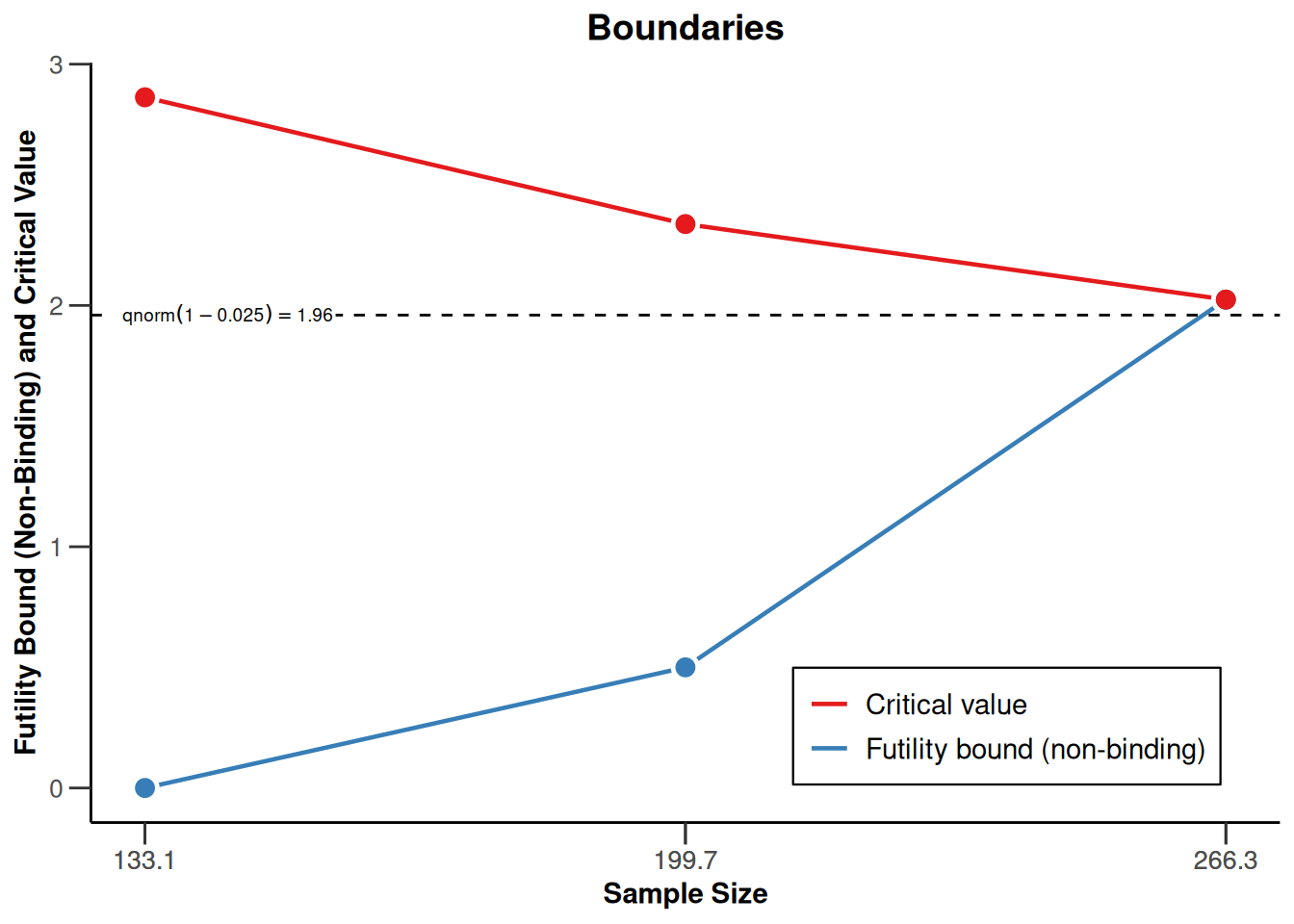On the effect size scale:

plot(r, type = 2)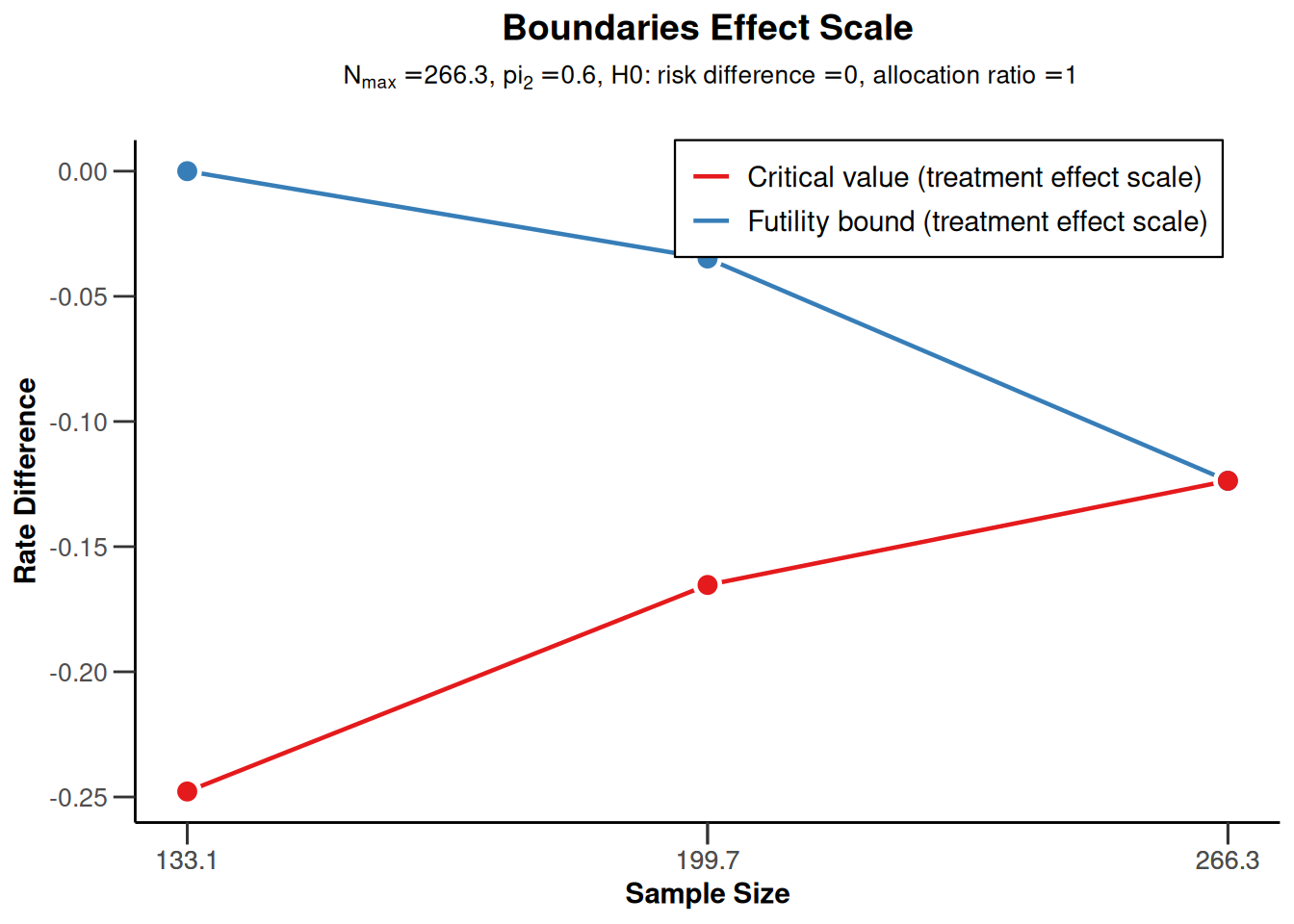On the $$p$$-value scale:

plot(r, type = 3)## Power assessment

Suppose that $$N = 280$$ subjects were planned for the study. The power if the failure rate in the active treatment group is $$\pi_1 = 0.40$$ or $$\pi_1 = 0.50$$ can be achieved as follows:

power <- getPowerRates(dGS,
maxNumberOfSubjects = 280,
pi1 = c(0.4, 0.5), pi2 = 0.6, directionUpper = FALSE
)
power$overallReject  0.914045 0.377853 Note that directionUpper = FALSE is used because the study is powered for alternatives $$\pi_1 - \pi_2$$ being smaller than 0. The power for $$\pi_1 = 0.50$$ (37.8%) is much reduced as compared to the case $$\pi_1 = 0.40$$ (where it exceeds 90%): ## Graphical illustration We also can graphically illustrate the power, the expected sample size, and the early stopping and futility stopping probabilities for a range of alternative values. This can be done by specifying the lower and the upper bound for $$\pi_1$$ in getPowerRates() and use the generic plot() command with type = 6: power <- getPowerRates(dGS, maxNumberOfSubjects = 280, pi1 = c(0.3, 0.6), pi2 = 0.6, directionUpper = FALSE ) plot(power, type = 6)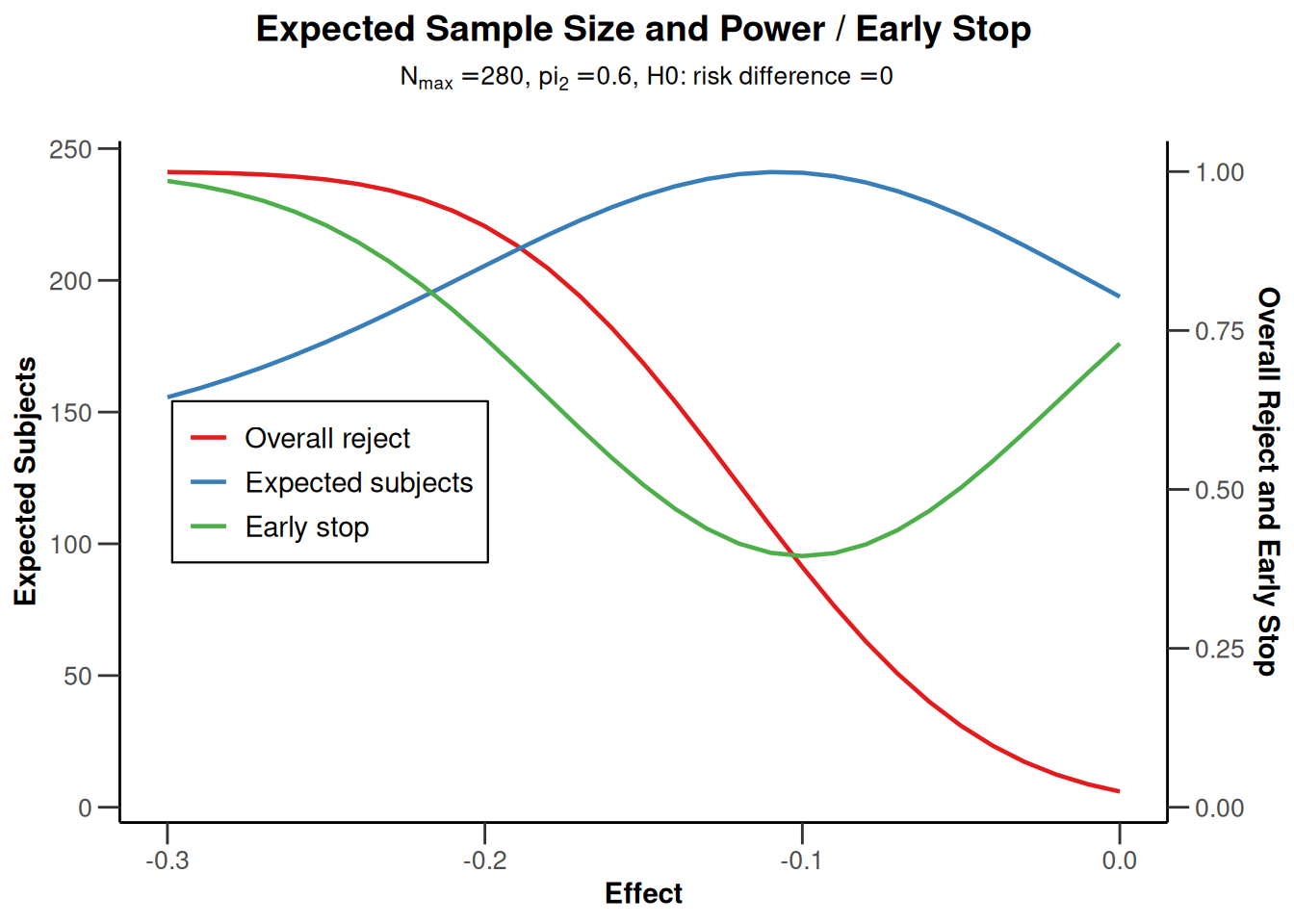# Sample size reassessment for testing rates Suppose that, using an adaptive design, the sample size from the above example can be increased in the last interim up to a 4-fold of the originally planned sample size for the last stage. Conditional power 90% based on the observed effect sizes (failure rates) should be used to increase the sample size. We want to use the inverse normal method to allow for the sample size increase and compare the test characteristics with the group sequential design from the above example. ## Assess power To assess the test characteristics of this adaptive design we first define the inverse normal design and then perform two simulations, one without and one with SSR: dIN <- getDesignInverseNormal( informationRates = c(0.5, 0.75, 1), alpha = 0.025, beta = 0.1, futilityBounds = c(0, 0.5) ) sim1 <- getSimulationRates(dIN, plannedSubjects = c(140, 210, 280), pi1 = seq(0.4, 0.5, 0.01), pi2 = 0.6, directionUpper = FALSE, maxNumberOfIterations = 1000, conditionalPower = 0.9, minNumberOfSubjectsPerStage = c(140, 70, 70), maxNumberOfSubjectsPerStage = c(140, 70, 70), seed = 1234 ) sim2 <- getSimulationRates(dIN, plannedSubjects = c(140, 210, 280), pi1 = seq(0.4, 0.5, 0.01), pi2 = 0.6, directionUpper = FALSE, maxNumberOfIterations = 1000, conditionalPower = 0.9, minNumberOfSubjectsPerStage = c(NA, 70, 70), maxNumberOfSubjectsPerStage = c(NA, 70, 4 * 70), seed = 1234 ) Note that the sample sizes will be calculated under the assumption that the conditional power for the subsequent stage is 90%. If the resulting sample size is larger, the upper bound (4*70 = 280) is used. Note also that sim1 can also be calculated using getPowerRates() or can also easier be simulated without specifying conditionalPower, minNumberOfSubjectsPerStage, and maxNumberOfSubjectsPerStage (which obviously is redundant for sim1) but this way ensures that the calculated objects sim1 and sim2 contain exactly the same parameters and therefore can easier be combined (see below). We can look at the power and the expected sample size of the two procedures and assess the power gain of using the adaptive design which comes along with an increased expected sample size: sim1$pi1
  0.40 0.41 0.42 0.43 0.44 0.45 0.46 0.47 0.48 0.49 0.50
round(sim1$overallReject, 3)   0.921 0.890 0.853 0.810 0.752 0.721 0.675 0.582 0.526 0.469 0.405 round(sim2$overallReject, 3)
  0.976 0.971 0.940 0.912 0.882 0.869 0.800 0.718 0.695 0.601 0.475
round(sim1$expectedNumberOfSubjects, 1)   202.1 209.3 216.4 219.5 222.0 230.9 231.1 238.3 234.2 237.9 236.5 round(sim2$expectedNumberOfSubjects, 1)
  240.7 251.6 270.6 278.8 286.6 305.8 323.8 330.9 336.4 336.8 349.3

## Illustrate power difference

We now want to graphically illustrate the gain in power when using the adaptive sample size recalculation. We use ggplot2 (see ggplot2.tidyverse.org) for doing this. First, a dataset df combining sim1and sim2 is defined with the additional variable SSR. Defining mytheme and using the following ggplot2 commands, the difference in power and ASN of the two strategies is illustrated. It shows that at least for (absolute) effect difference > 0.15 an overall power of more than around 85% can be achieved with the proposed sample size recalculation strategy.

library(ggplot2)

dataSim1 <- as.data.frame(sim1, niceColumnNamesEnabled = FALSE)
dataSim2 <- as.data.frame(sim2, niceColumnNamesEnabled = FALSE)

dataSim1$SSR <- rep("no SSR", nrow(dataSim1)) dataSim2$SSR <- rep("SSR", nrow(dataSim2))
df <- rbind(dataSim1, dataSim2)

myTheme <- theme(
axis.title.x = element_text(size = 12), axis.text.x = element_text(size = 12),
axis.title.y = element_text(size = 12), axis.text.y = element_text(size = 12),
plot.title = element_text(size = 14, hjust = 0.5),
plot.subtitle = element_text(size = 12, hjust = 0.5)
)

p <- ggplot(
data = df,
aes(x = effect, y = overallReject, group = SSR, color = SSR)
) +
geom_line(size = 1.1) +
geom_line(aes(
x = effect, y = expectedNumberOfSubjects / 400,
group = SSR, color = SSR
), size = 1.1, linetype = "dashed") +
scale_y_continuous("Power",
sec.axis = sec_axis(~ . * 400, name = "ASN"),
limits = c(0.2, 1)
) +
xlab("effect") +
ggtitle("Power and ASN", "Power solid, ASN dashed") +
geom_hline(size = 0.5, yintercept = 0.8, linetype = "dotted") +
geom_hline(size = 0.5, yintercept = 0.9, linetype = "dotted") +
geom_vline(size = 0.5, xintercept = c(-0.2, -0.15), linetype = "dashed") +
theme_classic() +
myTheme

plot(p)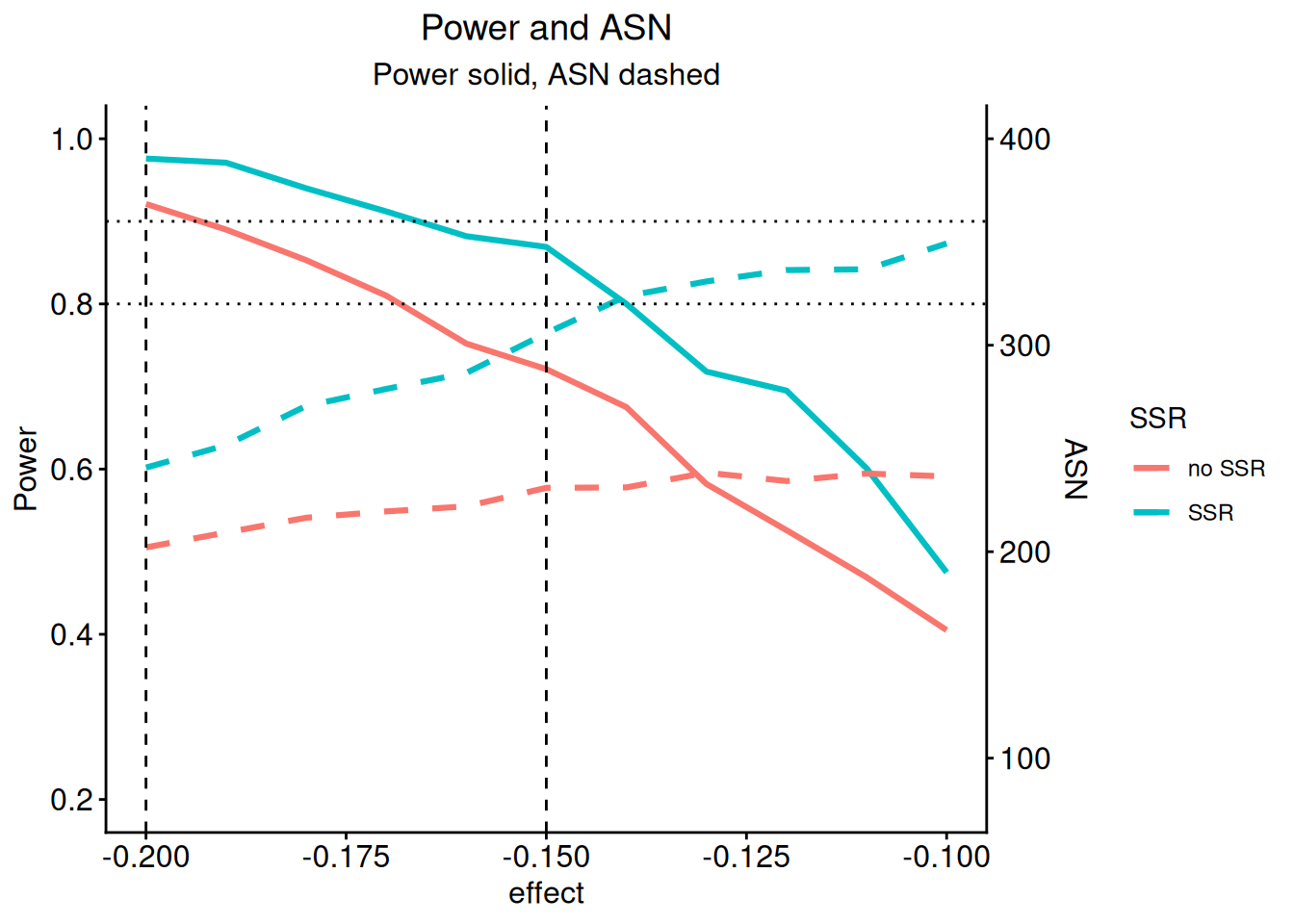For saving the graph, use

ggplot2::ggsave(filename = "c:/yourdirectory/comparison.png", plot = ggplot2::last_plot(), device = NULL, path = NULL, scale = 1.2, width = 20, height = 12, units = "cm", dpi = 600, limitsize = TRUE)

For another example of using ggplot2 in rpact see also the vignette Supplementing and enhancing rpact’s graphical capabilities with ggplot2.

## Histogram of sample sizes

Finally, we create a histogram for the attained sample size of the study when using the adaptive sample size recalculation.

With the getData() command the simulation results are obtained and str(simdata) provides information of the struture of this data:

simData <- getData(sim2)
str(simData)
'data.frame':   24579 obs. of  19 variables:
$iterationNumber : num 1 2 2 2 3 3 4 4 4 5 ...$ stageNumber              : num  1 1 2 3 1 2 1 2 3 1 ...
$pi1 : num 0.4 0.4 0.4 0.4 0.4 0.4 0.4 0.4 0.4 0.4 ...$ pi2                      : num  0.6 0.6 0.6 0.6 0.6 0.6 0.6 0.6 0.6 0.6 ...
$numberOfSubjects : num 140 140 70 147 140 70 140 70 91 140 ...$ numberOfCumulatedSubjects: num  140 140 210 357 140 210 140 210 301 140 ...
$rejectPerStage : num 1 0 0 1 0 1 0 0 1 1 ...$ futilityPerStage         : num  0 0 0 0 0 0 0 0 0 0 ...
$testStatistic : num 3.05 2.03 2.07 4.07 2.03 ...$ testStatisticsPerStage   : num  3.054 2.028 0.718 4.547 2.029 ...
$overallRate1 : num 0.329 0.414 0.438 0.369 0.429 ...$ overallRate2             : num  0.586 0.586 0.581 0.607 0.6 ...
$stagewiseRates1 : num 0.329 0.414 0.486 0.27 0.429 ...$ stagewiseRates2          : num  0.586 0.586 0.571 0.644 0.6 ...
$sampleSizesPerStage1 : num 70 70 35 74 70 35 70 35 46 70 ...$ sampleSizesPerStage2     : num  70 70 35 73 70 35 70 35 45 70 ...
$trialStop : logi TRUE FALSE FALSE TRUE FALSE TRUE ...$ conditionalPowerAchieved : num  NA NA 0.602 0.9 NA ...
$pValue : num 0.00112984 0.02126124 0.23628281 0.00000272 0.02121903 ... Depending on $$\pi_1$$ (in this example, for $$\pi_1 = 0.5$$), you can create the histogram of the simulated total sample size as follows: simDataPart <- simData[simData$pi1 == 0.5, ]

overallSampleSizes <-
sapply(1:1000, function(i) {
sum(simDataPart[simDataPart$iterationNumber == i, ]$numberOfSubjects)
})

hist(overallSampleSizes, main = "Histogram", xlab = "Achieved sample size")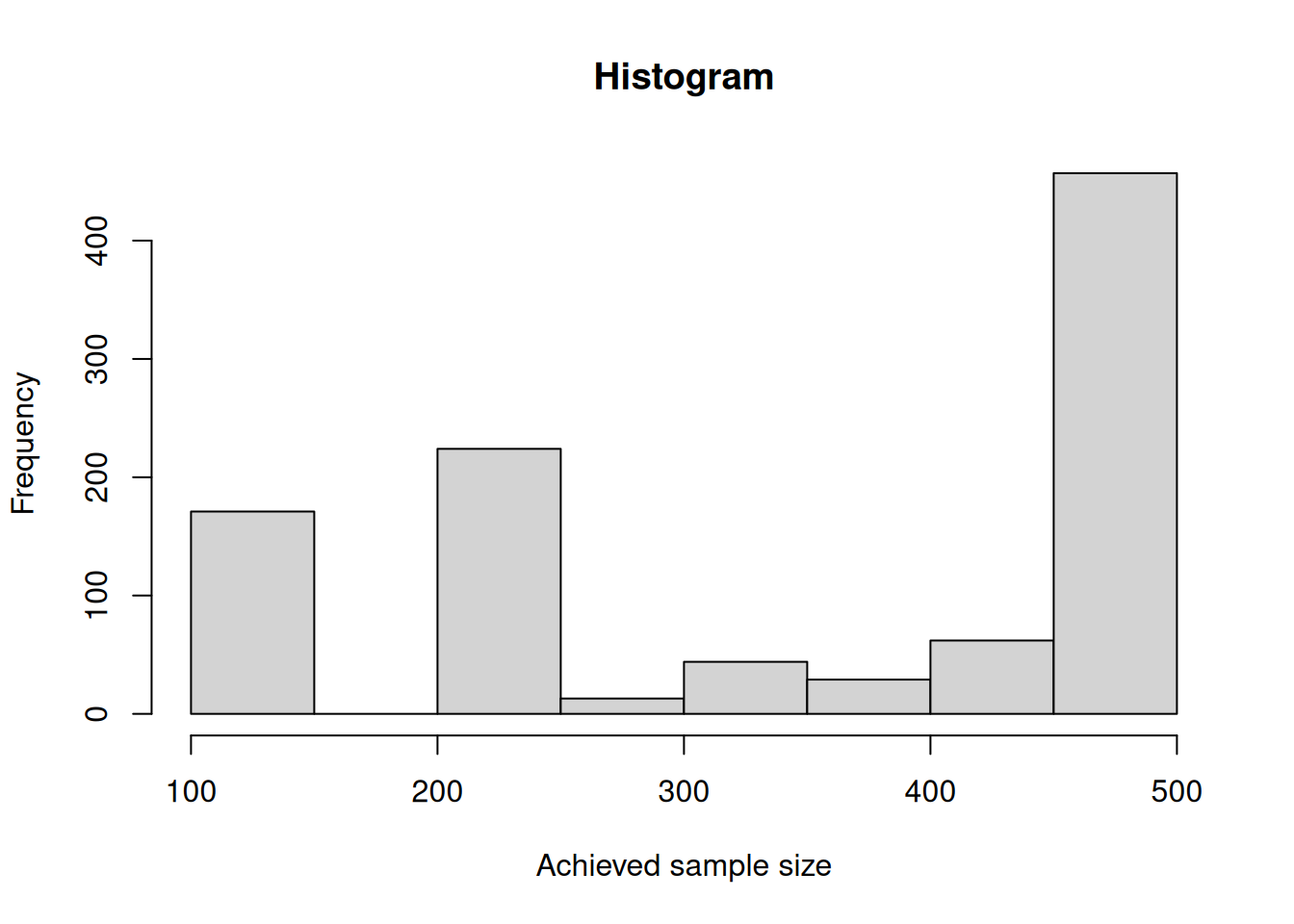How often the maximum and other sample sizes are reached over the stages can be obtained as follows:

subjectsRange <- cut(simDataPart$numberOfSubjects, c(69, 70, 139, 140, 210, 279, 280), labels = c( "(69,70]", "(70,139]", "(139,140]", "(140,210]", "(210,279]", "(279,280]" ) ) kable(round(prop.table(table(simDataPart$stageNumber, subjectsRange), margin = 1) * 100, 1))
(69,70] (70,139] (139,140] (140,210] (210,279] (279,280]
0 0.0 100.0 0.0 0.0 0.0
100 0.0 0.0 0.0 0.0 0.0
0 9.1 0.3 7.9 7.1 75.5

For this simulation, the originally planned sample size (70) was never selected for the third stage and in most of the cases the maximum of sample size (280) was used.